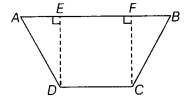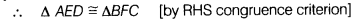# In the given figure, ABCD is a quadrilateral in which AD = BC, AB||CD

In the given figure, ABCD is a quadrilateral in which AD = BC, AB||CD and ∠ADC = ∠BCD. Show that the points A,B,C and D lie on a circle.To prove ABCD is a cyclic quadrilateral.
Construction Draw DE perpendicular to AB and CF perpendicular to AB.Proof In ∆AED and ∆BFC,
∠ AED = ∠BFC=90° [∵ DE ⊥ AB and CE ⊥ AB]
ED = FC
[ ∵ distance between two parallel lines are equal ]